# Stats Playbook: What is Anscombe’s Quartet and why is it important?

Stats Playbook: What is Anscombe’s Quartet and why is it important?

# Stats Playbook: What is Anscombe’s Quartet and why is it important?

The following paragraph is take from Wikipedia

“Anscombe’s quartet comprises four datasets that have nearly identical simple statistical properties, yet appear very different when graphed. Each dataset consists of eleven (x,y) points. They were constructed in 1973 by the statistician Francis Anscombe to demonstrate both the importance of graphing data before analyzing it and the effect of outliers on statistical properties”

Here is the famous Anscombe’s Quartet

``````library(xtable)

# Create the interactive table
ansombe.table <- xtable(anscombe)

print(ansombe.table, "html")
``````

x1 x2 x3 x4 y1 y2 y3 y4
1 10.00 10.00 10.00 8.00 8.04 9.14 7.46 6.58
2 8.00 8.00 8.00 8.00 6.95 8.14 6.77 5.76
3 13.00 13.00 13.00 8.00 7.58 8.74 12.74 7.71
4 9.00 9.00 9.00 8.00 8.81 8.77 7.11 8.84
5 11.00 11.00 11.00 8.00 8.33 9.26 7.81 8.47
6 14.00 14.00 14.00 8.00 9.96 8.10 8.84 7.04
7 6.00 6.00 6.00 8.00 7.24 6.13 6.08 5.25
8 4.00 4.00 4.00 19.00 4.26 3.10 5.39 12.50
9 12.00 12.00 12.00 8.00 10.84 9.13 8.15 5.56
10 7.00 7.00 7.00 8.00 4.82 7.26 6.42 7.91
11 5.00 5.00 5.00 8.00 5.68 4.74 5.73 6.89

Here it how it looks like graphically

``````library(ggplot2)

anscombe.1 <- data.frame(x = anscombe[["x1"]], y = anscombe[["y1"]], Set = "Anscombe Set 1")
anscombe.2 <- data.frame(x = anscombe[["x2"]], y = anscombe[["y2"]], Set = "Anscombe Set 2")
anscombe.3 <- data.frame(x = anscombe[["x3"]], y = anscombe[["y3"]], Set = "Anscombe Set 3")
anscombe.4 <- data.frame(x = anscombe[["x4"]], y = anscombe[["y4"]], Set = "Anscombe Set 4")

anscombe.data <- rbind(anscombe.1, anscombe.2, anscombe.3, anscombe.4)

gg <- ggplot(anscombe.data, aes(x = x, y = y))
gg <- gg + geom_point(color = "black")
gg <- gg + facet_wrap(~Set, ncol = 2)
gg
``````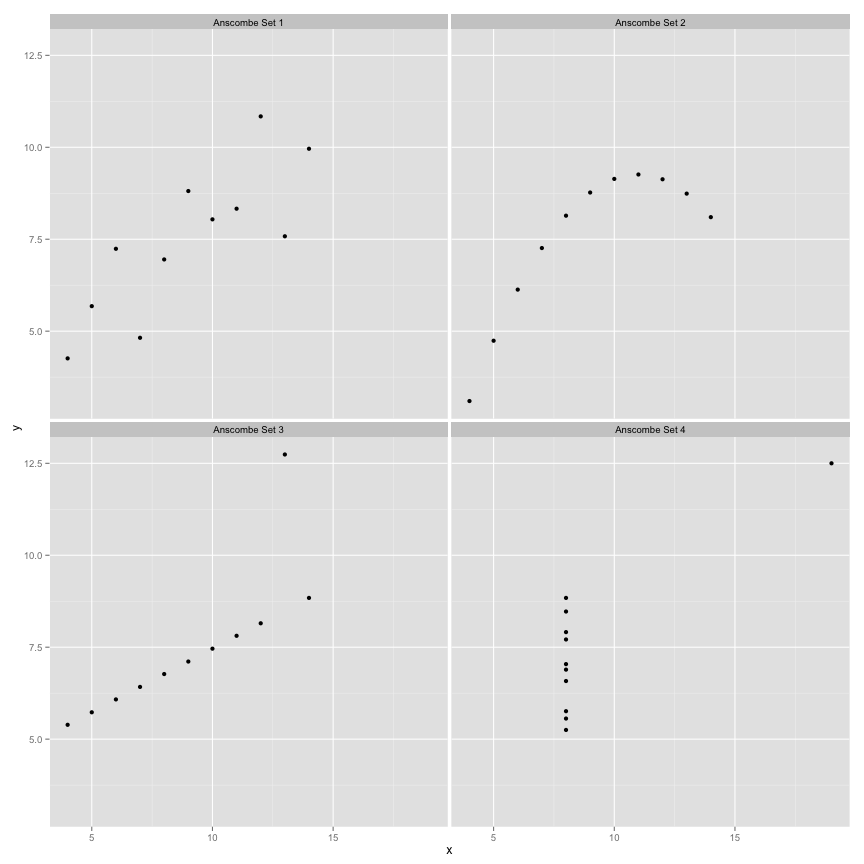Let’s do the simple descriptive statistics on each data set

Here is mean of x and y

``````aggregate(cbind(x, y) ~ Set, anscombe.data, mean)
``````
``````##              Set x     y
## 1 Anscombe Set 1 9 7.501
## 2 Anscombe Set 2 9 7.501
## 3 Anscombe Set 3 9 7.500
## 4 Anscombe Set 4 9 7.501
``````

And SD

``````aggregate(cbind(x, y) ~ Set, anscombe.data, sd)
``````
``````##              Set     x     y
## 1 Anscombe Set 1 3.317 2.032
## 2 Anscombe Set 2 3.317 2.032
## 3 Anscombe Set 3 3.317 2.030
## 4 Anscombe Set 4 3.317 2.031
``````

And correlation between x and y

``````library(plyr)

correlation <- function(data) {
x <- data.frame(r = cor(data\$x, data\$y))
return(x)
}

ddply(.data = anscombe.data, .variables = "Set", .fun = correlation)
``````
``````##              Set      r
## 1 Anscombe Set 1 0.8164
## 2 Anscombe Set 2 0.8162
## 3 Anscombe Set 3 0.8163
## 4 Anscombe Set 4 0.8165
``````

As can be seen they are pretty much the same for every data set.

Let’s perform linear regression model for each

``````model1 <- lm(y ~ x, subset(anscombe.data, Set == "Anscombe Set 1"))
model2 <- lm(y ~ x, subset(anscombe.data, Set == "Anscombe Set 2"))
model3 <- lm(y ~ x, subset(anscombe.data, Set == "Anscombe Set 3"))
model4 <- lm(y ~ x, subset(anscombe.data, Set == "Anscombe Set 4"))
``````

Here are the summaries

``````summary(model1)
``````
``````##
## Call:
## lm(formula = y ~ x, data = subset(anscombe.data, Set == "Anscombe Set 1"))
##
## Residuals:
##     Min      1Q  Median      3Q     Max
## -1.9213 -0.4558 -0.0414  0.7094  1.8388
##
## Coefficients:
##             Estimate Std. Error t value Pr(>|t|)
## (Intercept)    3.000      1.125    2.67   0.0257 *
## x              0.500      0.118    4.24   0.0022 **
## ---
## Signif. codes:  0 '***' 0.001 '**' 0.01 '*' 0.05 '.' 0.1 ' ' 1
##
## Residual standard error: 1.24 on 9 degrees of freedom
## Multiple R-squared:  0.667,  Adjusted R-squared:  0.629
## F-statistic:   18 on 1 and 9 DF,  p-value: 0.00217
``````
``````summary(model2)
``````
``````##
## Call:
## lm(formula = y ~ x, data = subset(anscombe.data, Set == "Anscombe Set 2"))
##
## Residuals:
##    Min     1Q Median     3Q    Max
## -1.901 -0.761  0.129  0.949  1.269
##
## Coefficients:
##             Estimate Std. Error t value Pr(>|t|)
## (Intercept)    3.001      1.125    2.67   0.0258 *
## x              0.500      0.118    4.24   0.0022 **
## ---
## Signif. codes:  0 '***' 0.001 '**' 0.01 '*' 0.05 '.' 0.1 ' ' 1
##
## Residual standard error: 1.24 on 9 degrees of freedom
## Multiple R-squared:  0.666,  Adjusted R-squared:  0.629
## F-statistic:   18 on 1 and 9 DF,  p-value: 0.00218
``````
``````summary(model3)
``````
``````##
## Call:
## lm(formula = y ~ x, data = subset(anscombe.data, Set == "Anscombe Set 3"))
##
## Residuals:
##    Min     1Q Median     3Q    Max
## -1.159 -0.615 -0.230  0.154  3.241
##
## Coefficients:
##             Estimate Std. Error t value Pr(>|t|)
## (Intercept)    3.002      1.124    2.67   0.0256 *
## x              0.500      0.118    4.24   0.0022 **
## ---
## Signif. codes:  0 '***' 0.001 '**' 0.01 '*' 0.05 '.' 0.1 ' ' 1
##
## Residual standard error: 1.24 on 9 degrees of freedom
## Multiple R-squared:  0.666,  Adjusted R-squared:  0.629
## F-statistic:   18 on 1 and 9 DF,  p-value: 0.00218
``````
``````summary(model4)
``````
``````##
## Call:
## lm(formula = y ~ x, data = subset(anscombe.data, Set == "Anscombe Set 4"))
##
## Residuals:
##    Min     1Q Median     3Q    Max
## -1.751 -0.831  0.000  0.809  1.839
##
## Coefficients:
##             Estimate Std. Error t value Pr(>|t|)
## (Intercept)    3.002      1.124    2.67   0.0256 *
## x              0.500      0.118    4.24   0.0022 **
## ---
## Signif. codes:  0 '***' 0.001 '**' 0.01 '*' 0.05 '.' 0.1 ' ' 1
##
## Residual standard error: 1.24 on 9 degrees of freedom
## Multiple R-squared:  0.667,  Adjusted R-squared:  0.63
## F-statistic:   18 on 1 and 9 DF,  p-value: 0.00216
``````

And graphically

``````gg <- gg + geom_smooth(formula = y ~ x, method = "lm", se = FALSE, data = anscombe.data)
gg
``````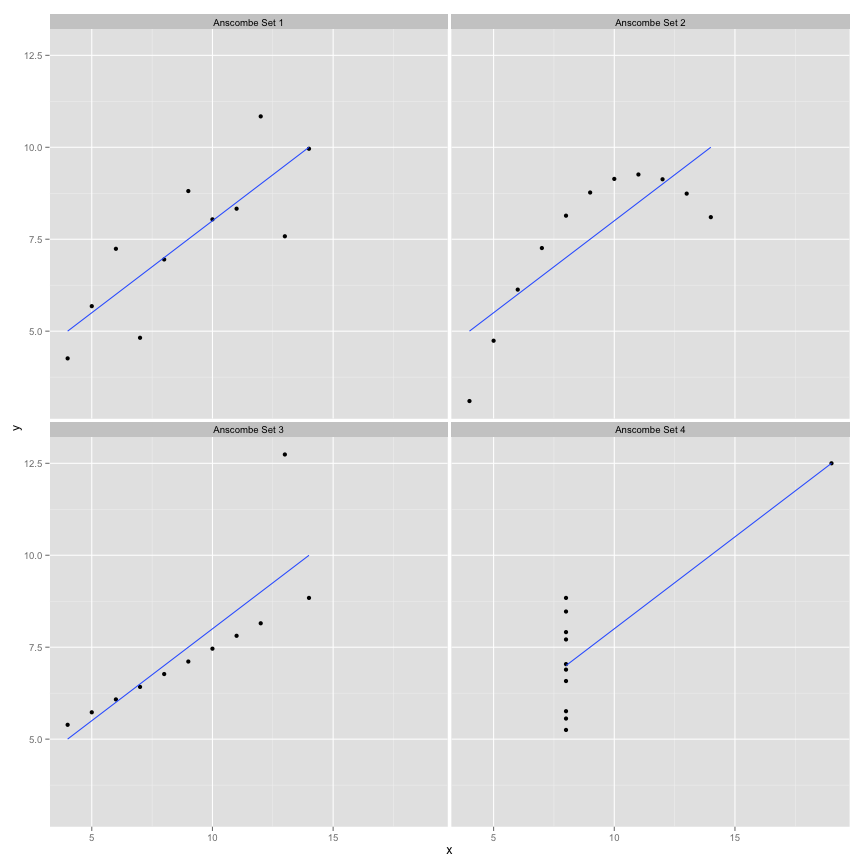It can be seen both graphically and from regression summary that each data set resulted in same statistical model!

Intercepts, coeficients and their p values are the same. SEE (standard error of the estimate, or SD of residuals), F-value and it’s p values are the same.

What is the conclusion: ALWAYS plot your data! And always do model diagnostics by plotting the residuals.

``````par(mfrow = c(2, 2))
plot(model1, main = "Model 1")
``````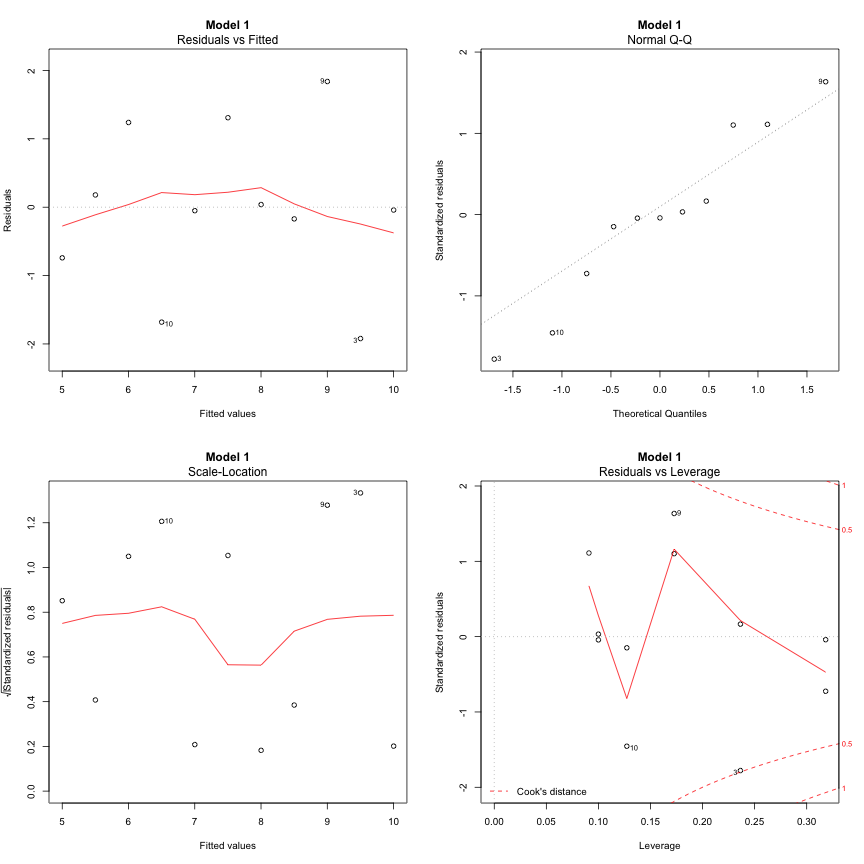``````plot(model2, main = "Model 2")
``````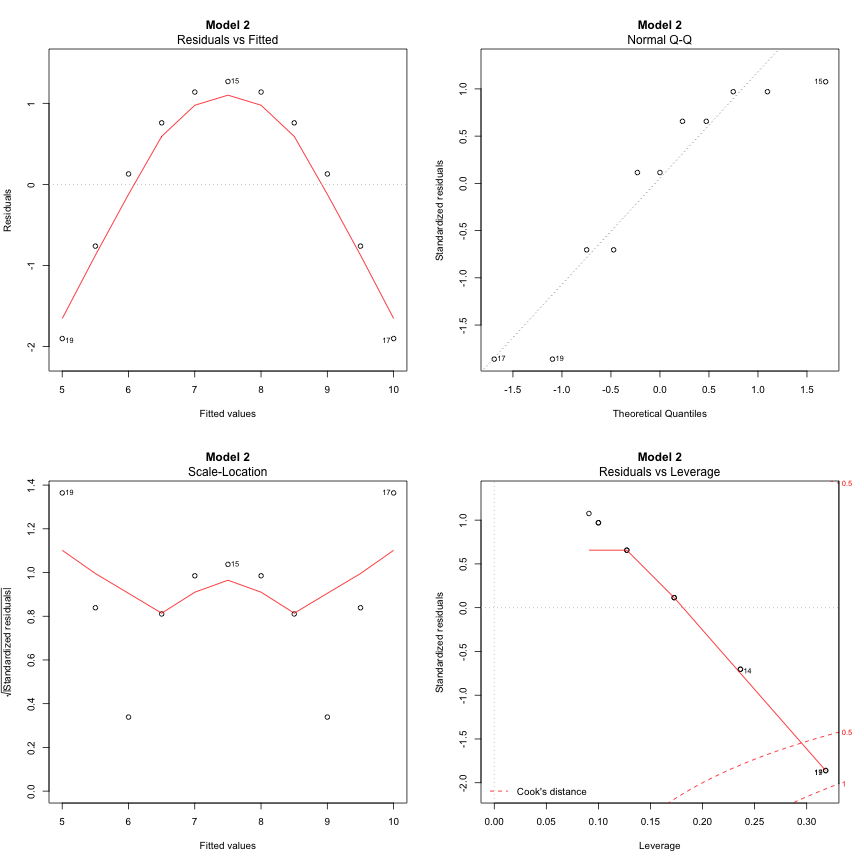``````plot(model3, main = "Model 3")
``````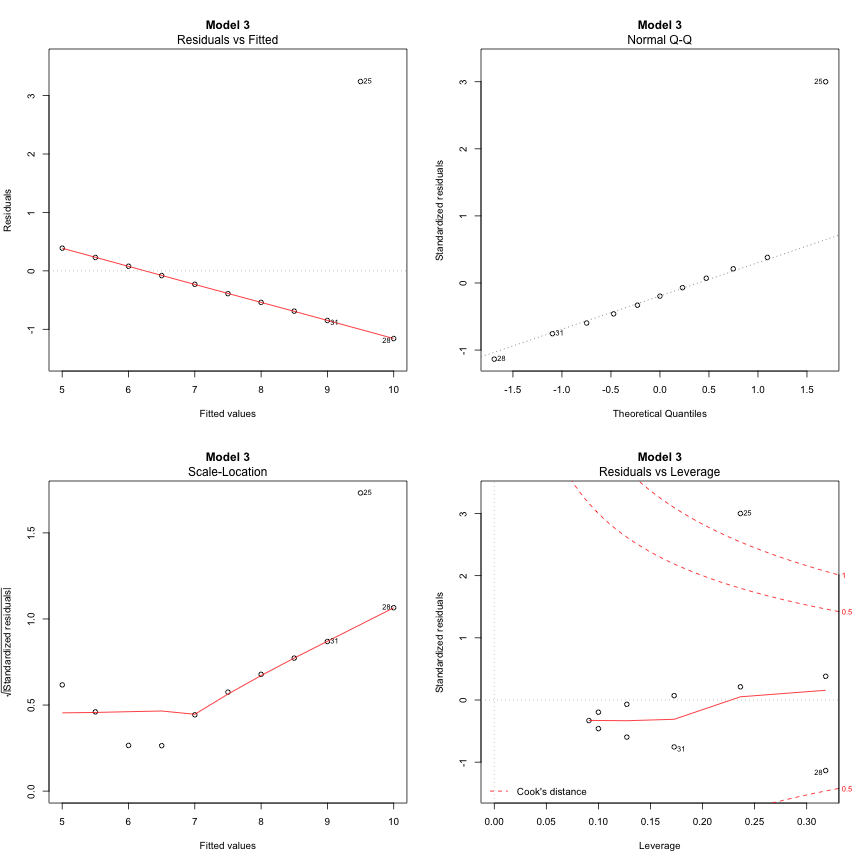``````plot(model4, main = "Model 4")
``````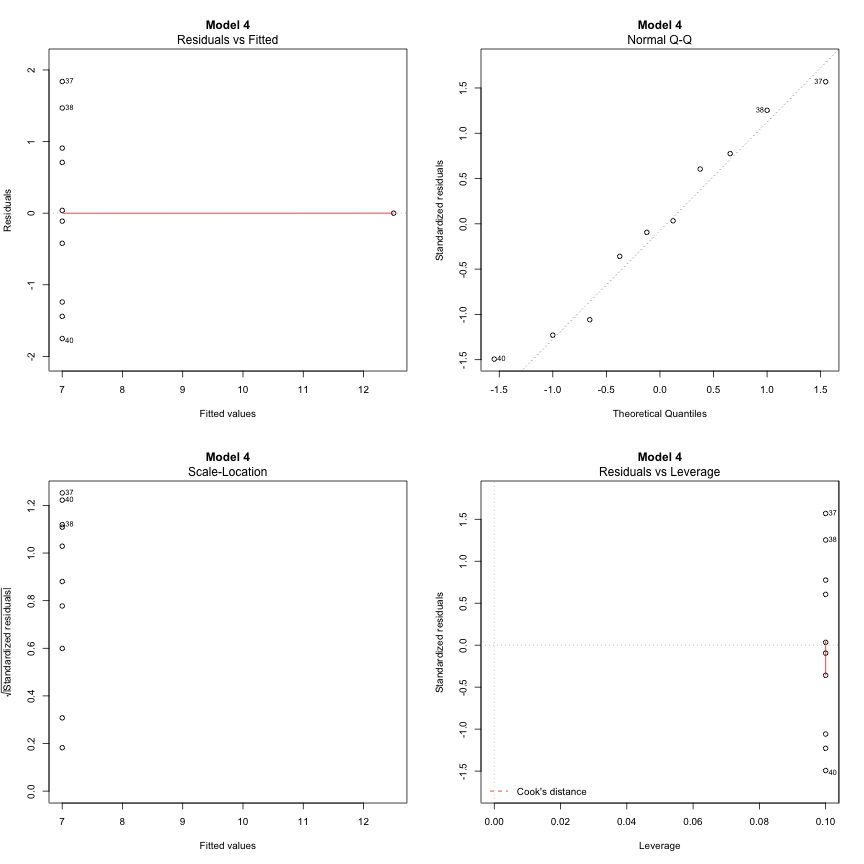In the next part I will be covering outliers and influential cases and their differenceI am a physical preparation coach from Belgrade, Serbia, grew up in Pula, Croatia (which I consider my home town). I was involved in physical preparation of professional, amateur and recreational athletes of various ages in sports such as basketball, soccer, volleyball, martial arts and tennis. Read More »

# Don't Miss Out on Fantastic Job Opportunities!

Be the first to find out about the newest & hottest sports jobs on the market.